# `20180516a` - Testing to format the data for experiments to be easily reproduced

The objective of this experiment is to streamkine data acquisition

# Images of the Experiment

## Others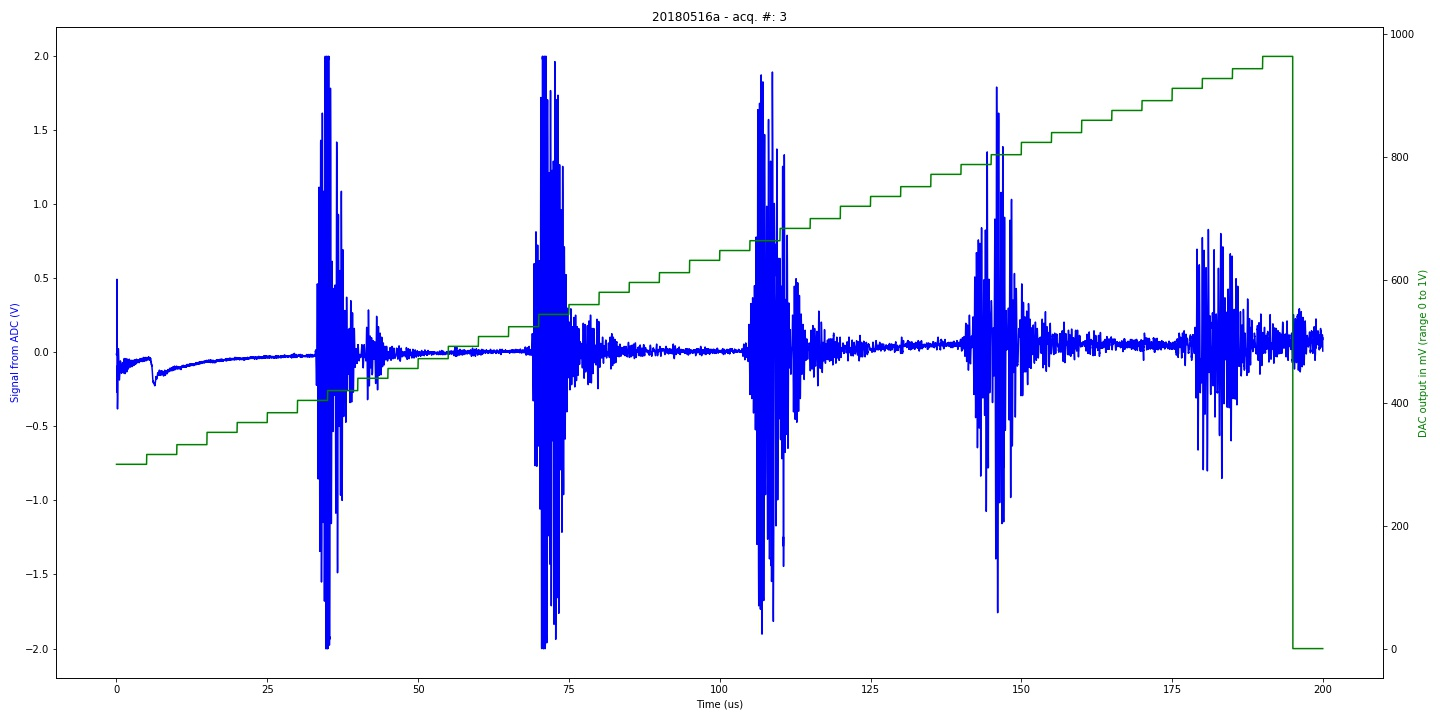FFT of the of 20180516a experiment. Classical experiment with calibration piezo (category: FFT).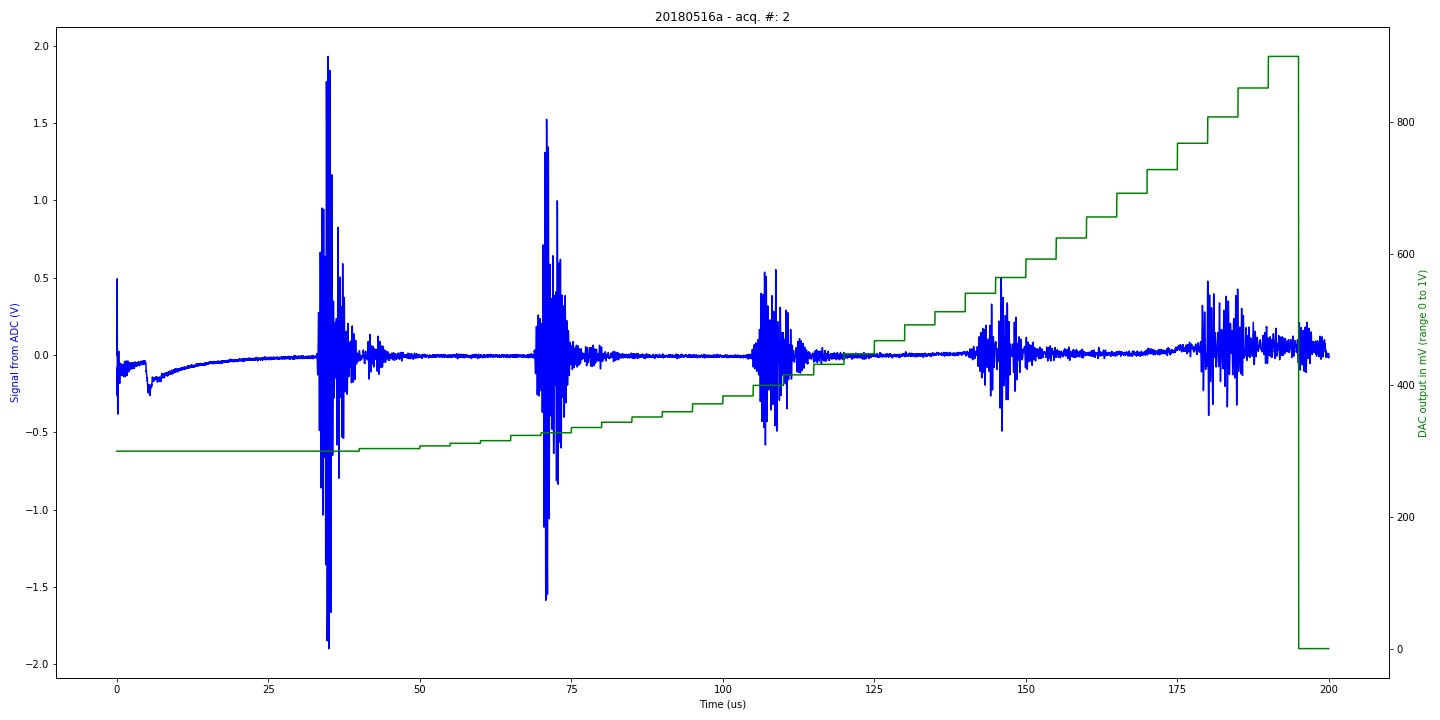FFT of the of 20180516a experiment. Classical experiment with calibration piezo (category: FFT).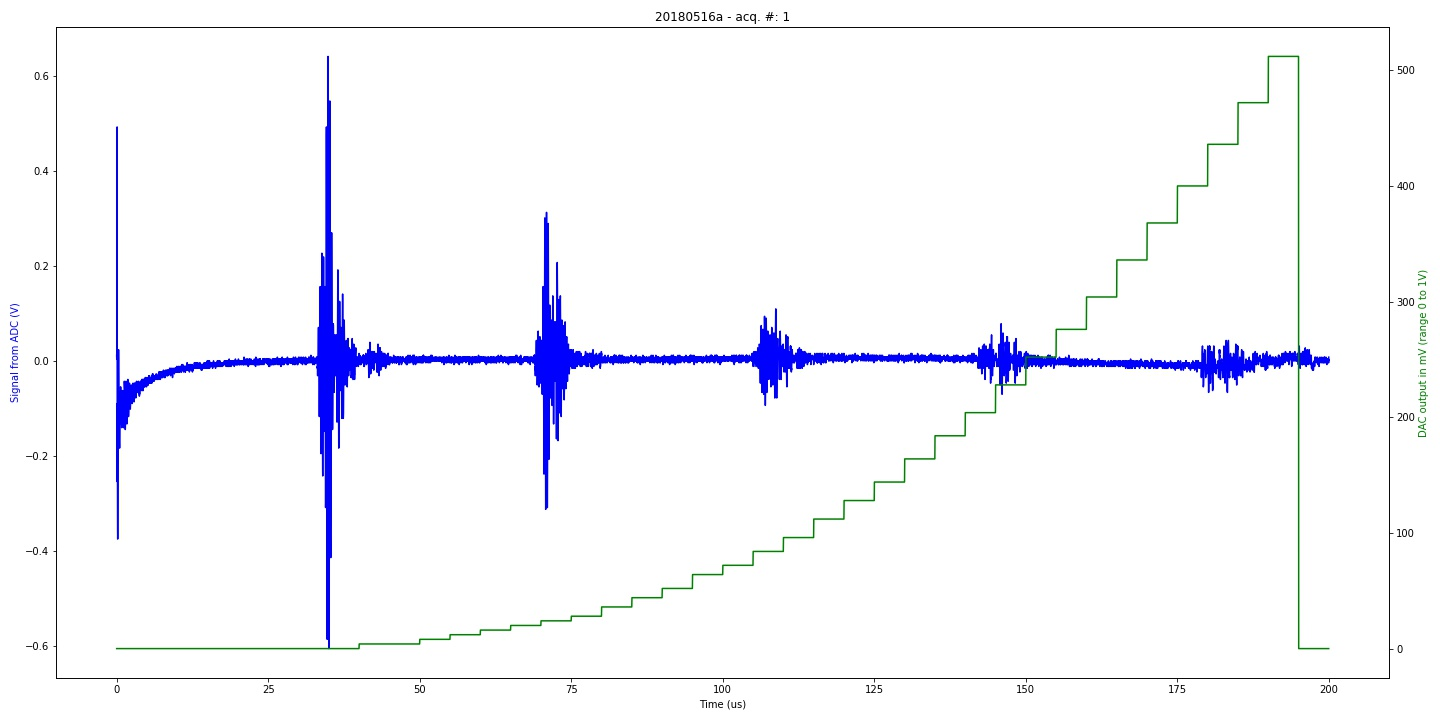FFT of the of 20180516a experiment. Classical experiment with calibration piezo (category: FFT).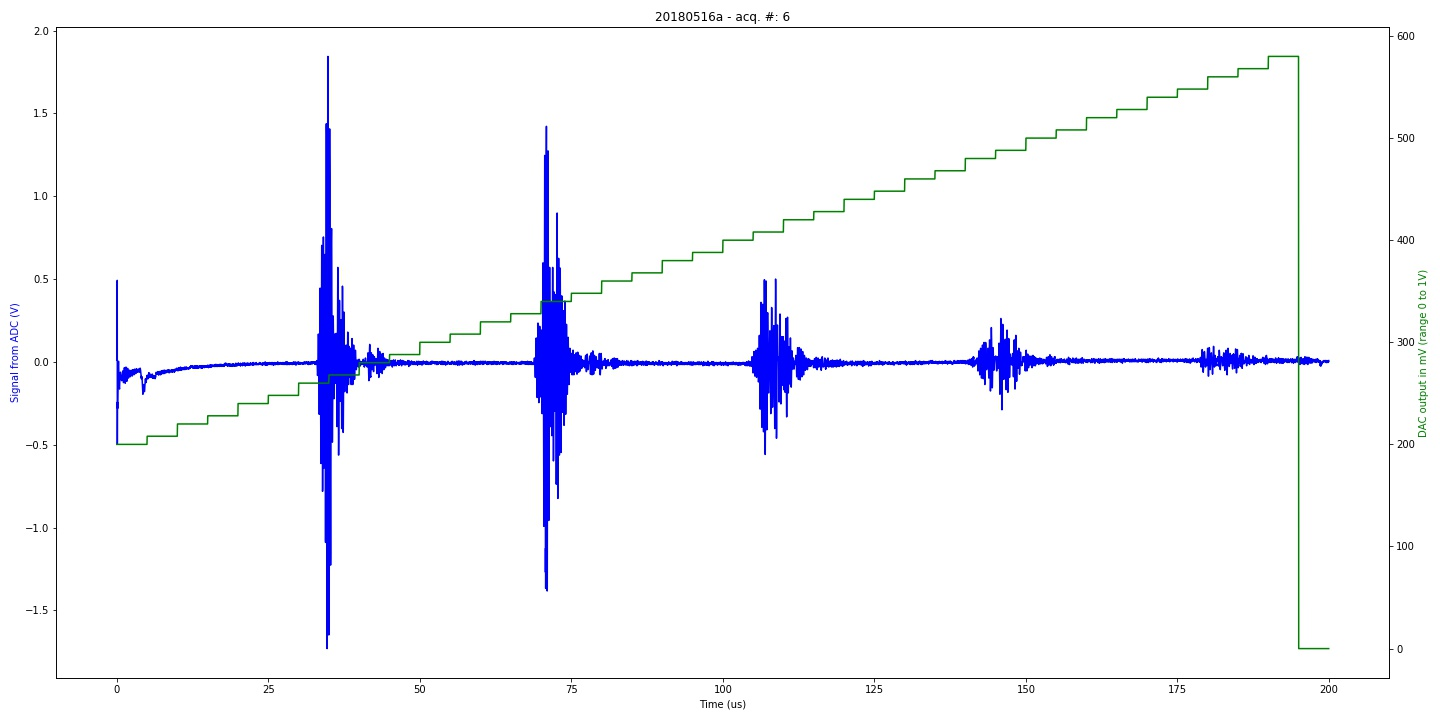Graph of 20180516a experiment. Classical experiment with calibration piezo (category: graph).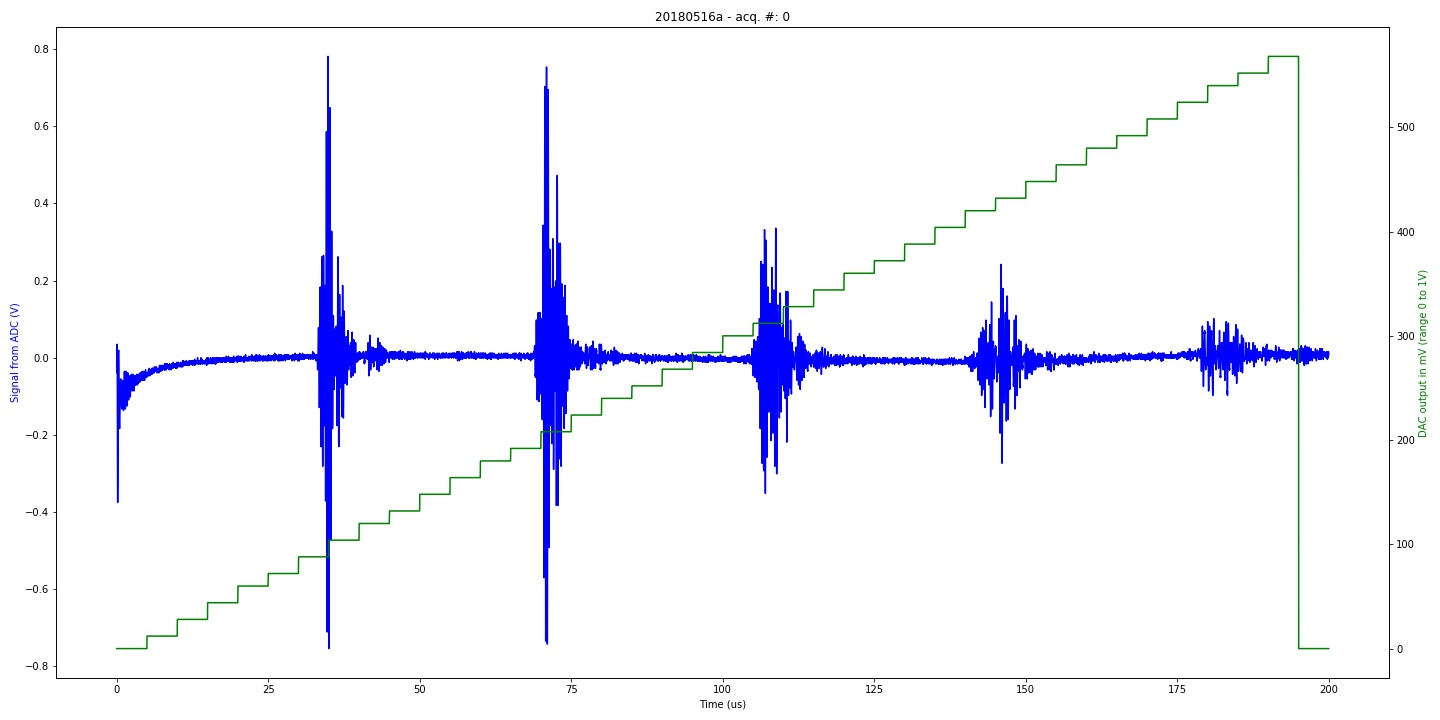Graph of 20180516a experiment. Classical experiment with calibration piezo (category: graph).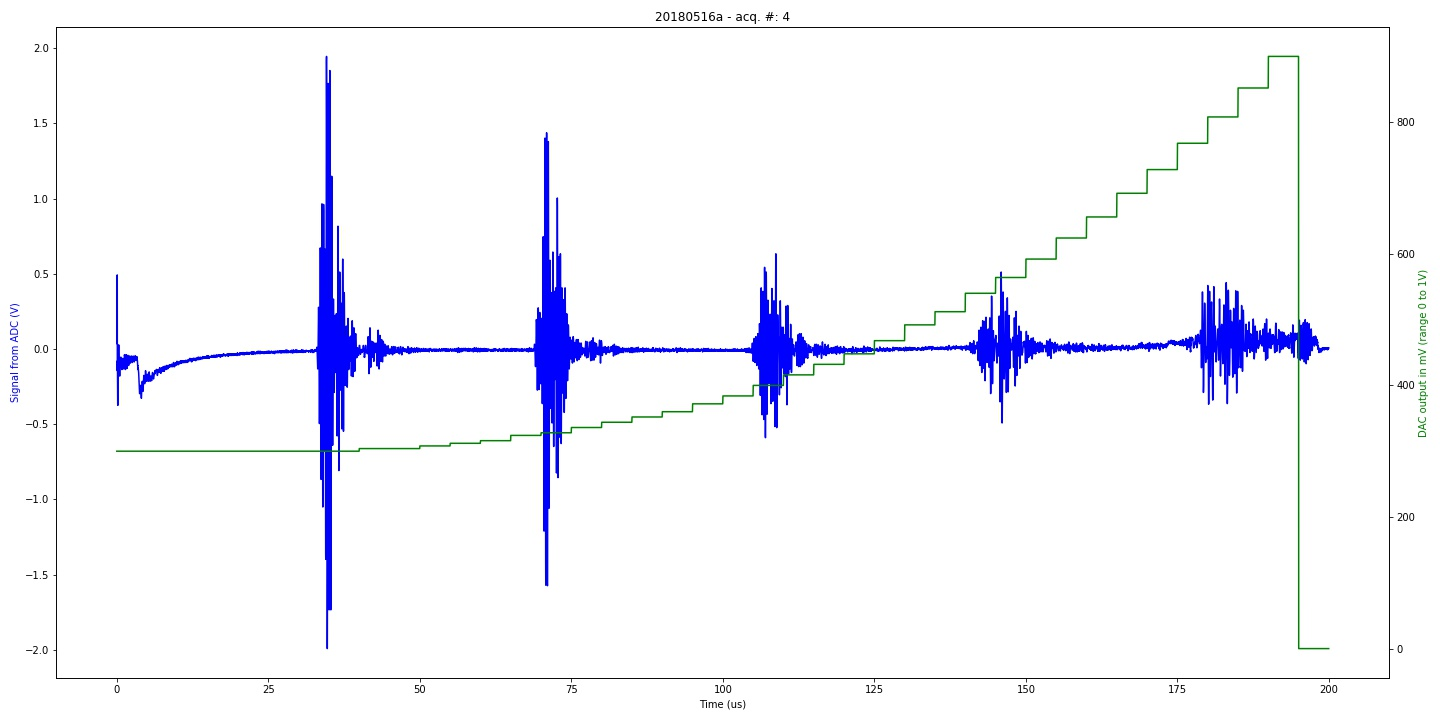Graph of 20180516a experiment. Classical experiment with calibration piezo (category: graph).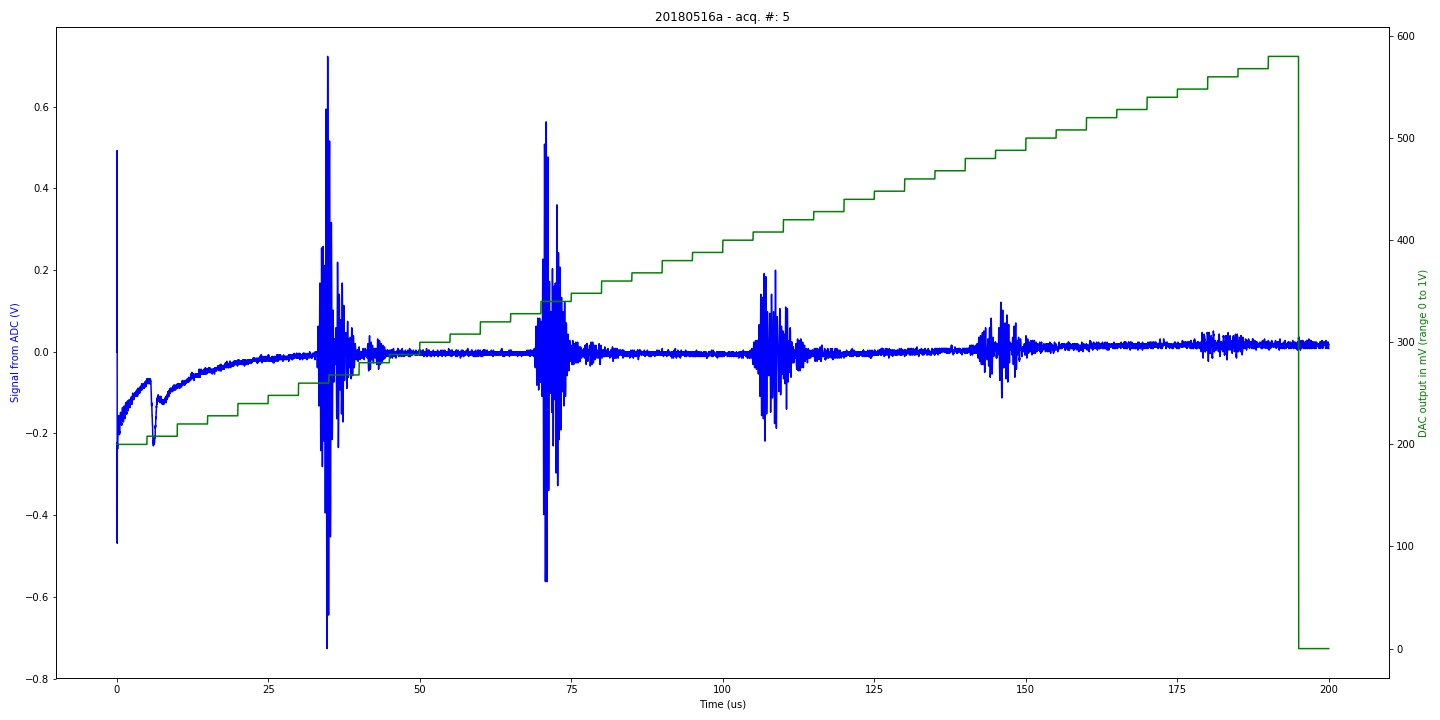Graph of 20180516a experiment. Classical experiment with calibration piezo (category: graph).